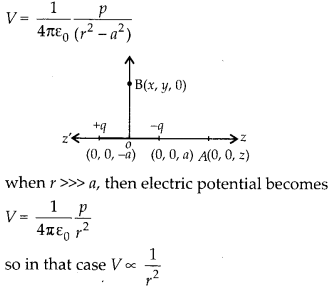Enlightened

# Question 21: NCERT Solutions for 12th Class Physics: Chapter 2-Electrostatic Potential and Capacitance

• 0

Question 21: NCERT Solutions for 12th Class Physics: Chapter 2-Electrostatic Potential and Capacitance

Two charges -q and +q are located at points (0,0, -a) and (0,0, a), respectively.

(a) What is the electrostatic potential at the points (0,0, z) and (x, y, 0)?
(b) Obtain the dependence of potential on the distance r of a point from the origin when r/a »1.
(c) How much work is done in moving a small test charge from the point (5, 0, 0) to (-7, 0, 0) along the x-axis? Does the

answer change if the path of the test charge between the some points is not along the x-axis?

Share

1. Solution:
(a) The two point charges form an electric dipole of moment p = q 2a directed along + z-axis. Point A (0, 0, z) lies on the axis of electric dipole, so electric potential at point A isPoint B (x, y, 0) lies on the equatorial plane of electric dipole, so electric potential at point B is zero i.e. VB = 0
(b) Electric potential at any point on the axis of electric dipole at distance r from its centre is(c) As both the points C (5, 0, 0) and D (-7, 0, 0) lie on the perpendicular bisector of electric dipole, so electric potential at both the points is zero. Hence work done in moving the charge from C to D is
WCD = q0 [VD – VC] × O or WCD = O
This work done will remain equal to zero even if the path of the test charge between the same points is changed, as electric field is conservative field and work done in moving a charge between the two points in electric field is independent of the path chosen to move the charge.

Check the complete chapter with solutions.

NCERT Solutions for 12th Class Physics: Chapter 2-Electrostatic Potential and Capacitance

• 0